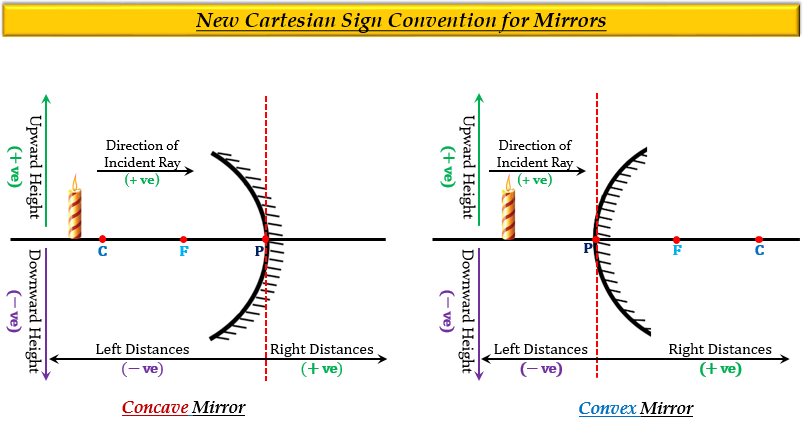# According to New Cartesian Sign Convention:(a) focal length of concave mirror is positive and that of convex mirror is negative(b) focal length of both concave and convex mirrors is positive(c) focal length of both concave and convex mirrors is negative(d) focal length of concave mirror is negative and that of convex mirror is positive

(d) focal length of concave mirror is negative and that of convex mirror is positive

Explanation

The focal length of a concave mirror is negative, and that of a convex mirror is positive.

This is because according to the New Cartesian Sign Convention, the distances on the left side of the mirror from the pole (in front of the mirror) are given a negative sign, and in the concave mirror the focus lies in front of the mirror. On the other hand, distances on the right side of the mirror from the pole (behind the mirror) are given a positive sign, and in the convex mirror, the focus lies behind the mirror.Updated on: 10-Oct-2022

422 Views